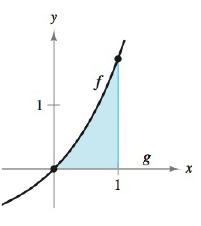Chapter 5.5, Problem 5E### Calculus: An Applied Approach (Min...

10th Edition
Ron Larson
ISBN: 9781305860919

#### Solutions

Chapter
Section### Calculus: An Applied Approach (Min...

10th Edition
Ron Larson
ISBN: 9781305860919
Textbook Problem
1 views

# Finding the Area Bounded by Two Graphs In Exercises 1-8, find the area of the region. See Examples 1, 2, 3, and 4. f ( x ) = e x − 1 g ( x ) = 0To determine

To calculate: The area bounded by the region f(x)=ex1 and g(x)=0 in the provided figure,Explanation

Given Information:

The provided function is f(x)=ex1 and g(x)=0 and the provided graph is shown below,

Formula used:

The function f(x) and g(x) are the continuous function on interval [a,b] and g(x)f(x) for all x in [a,b], then, the area bounded by the graphs of f(x) and g(x),x=a and x=b is,

A=ab[f(x)g(x)]dx

Calculation:

Consider the provided function is f(x)=ex1 and g(x)=0.

In the provided function f(x)=ex1 and g(x)=0 where, g(x)f(x)

### Still sussing out bartleby?

Check out a sample textbook solution.

See a sample solution

#### The Solution to Your Study Problems

Bartleby provides explanations to thousands of textbook problems written by our experts, many with advanced degrees!

Get Started

#### Show that sin x x if 0 x 2.

Single Variable Calculus: Early Transcendentals

#### True or False: , for –π ≤ t ≤ π, is smooth.

Study Guide for Stewart's Multivariable Calculus, 8th

#### What is the partial fraction form of ?

Study Guide for Stewart's Single Variable Calculus: Early Transcendentals, 8th